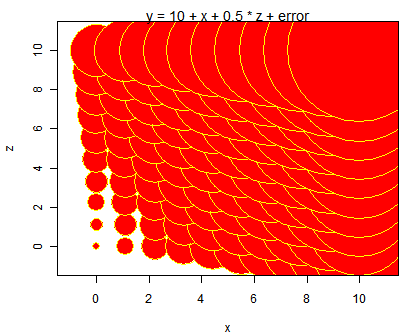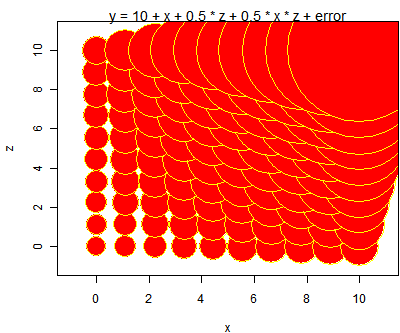# To Observe Interactions in Bubble Plots

### Yihui Xie 2008-09-18

Is it clear enough to observe the interaction between `z` and `x` from these bubble plots?If there is no interaction between `x` and `z`, the size of bubbles will increase at the same rate while either `x` or `z` is fixed.But when interaction exists, the rate of increasing will be different.

R code:

``````sq = 1:10
x = rep(sq, 10)
z = rep(sq, each = 10)
y = c(outer(sq, sq, function(x, z) 10 + 1 * x + 0.5 *
z + 0.5 * x * z + runif(1)))
symbols(x, z, y, bg = "red", fg = "yellow",
main = "y = 10 + x + 0.5 * z + 0.5 * x * z + error")
y = c(outer(sq, sq, function(x, z) 1 * x + 0.5 * z + runif(1)))
symbols(x, z, y, bg = "red", fg = "yellow",
main = "y = 10 + x + 0.5 * z + error")
``````